## Proof That the Equation of Motion For an Elastic Isotropic Material is a Wave Equation

A material is elastic if it returns to its original shape when a force is removed and isotropic if the material has no favoured direction for an applied force.

Let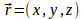and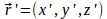be the position vectors of a point P before and after deformation. The displacement of the point P is then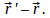If this displacement is small then the force will be proportional to the displacement.

Let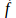be the force density, then the motion of the material is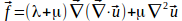where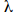and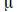are constants of the material.

Neglecting any body forces such as gravity,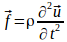where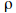is the density of the material.

Hence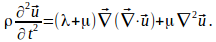Any vector field can be written as the sum of solenoidal and irrotational fields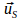and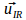satisfying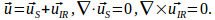Hence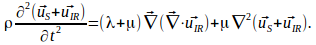Take the divergence of this equation to give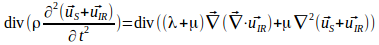Interchange the order of differentiation on the left and use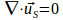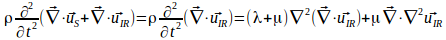Move everything to the left and factor out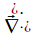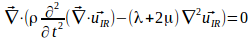Since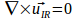the curl of the expression in brackets is zero. The only way the curl and divergence can both be zero is if the expression is zero hence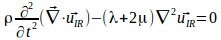The wave equation takes this form.

Take the curl of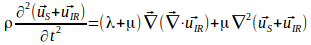to give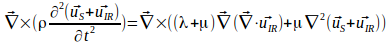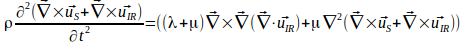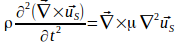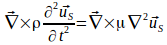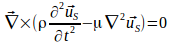Hence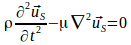This also has the form of a wave equation.